# Temperature and Humidity Sensor Controlling Humidifier

The use of a simple circuit and a DHT11 sensor to read the temperature and humidity. Allowing for the automated control of a humidifier.

IntermediateFull instructions provided7 hours1,779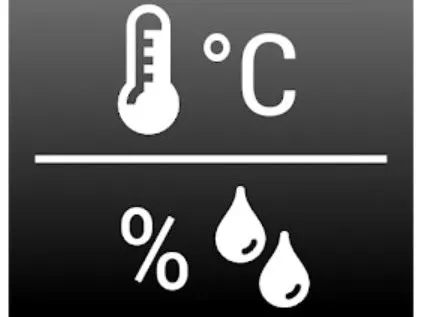## Things used in this project

### Hardware componentsParticle Photon
×2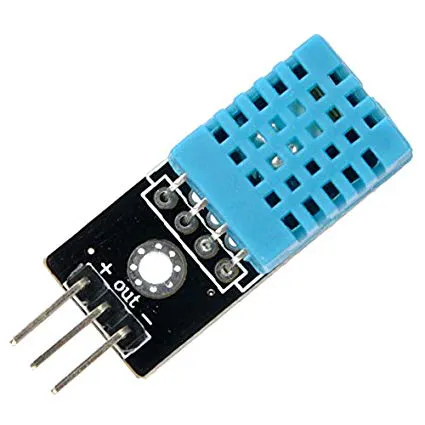DHT11 Temperature & Humidity Sensor (3 pins)
×1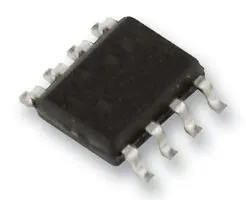Operational Amplifier, Op Amp + Comparator + Reference
×1Resistor 10k ohm
×2LED (generic)
×2Jumper wires (generic)
×10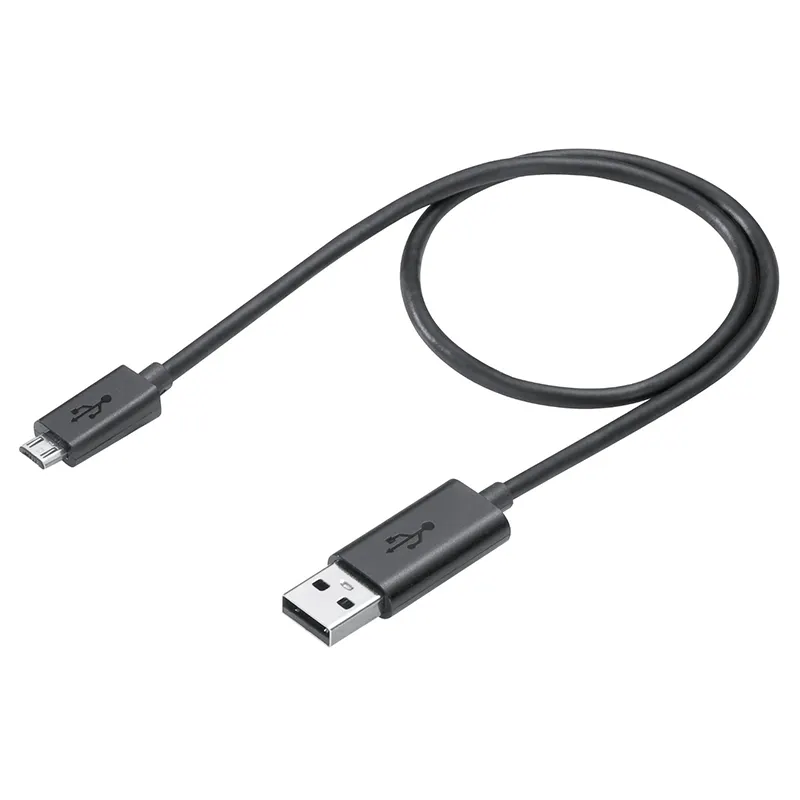Micro-USB to USB Cable (Generic)
×2

### Software apps and online servicesParticle Build Web IDEThingSpeak API

## Schematics

### The DHT11 Sensor Circuit

There was no DHT11 part available in the program so I used a different temperature sensor. However, I only used the three pins exactly how I would with the DHT11 that we used.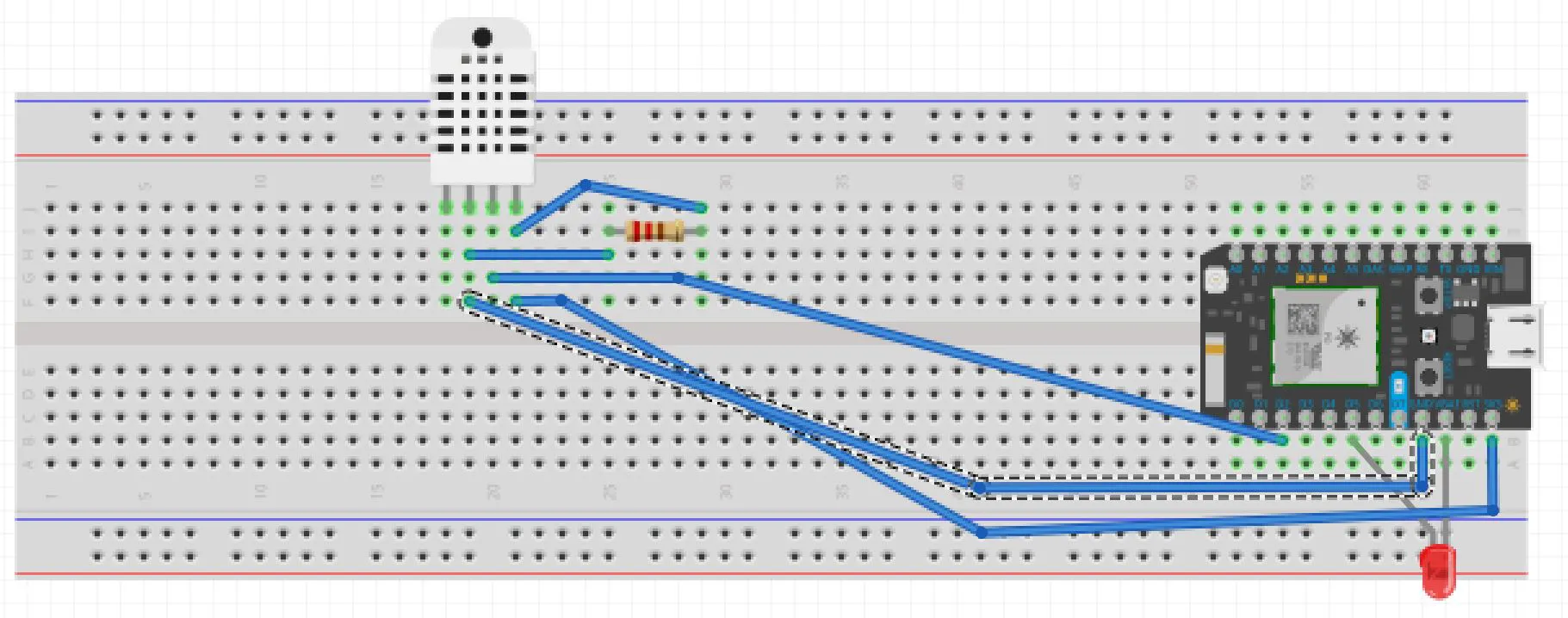### Humidifier Sensor Circuit

All of the wires going off of the breadboard are connected to our hand-made humidifier. These wires may vary for a different humidifier. The black node and the resistor between the photon and the op-amp is not needed for the completion of the project. It was an unneccesary add on.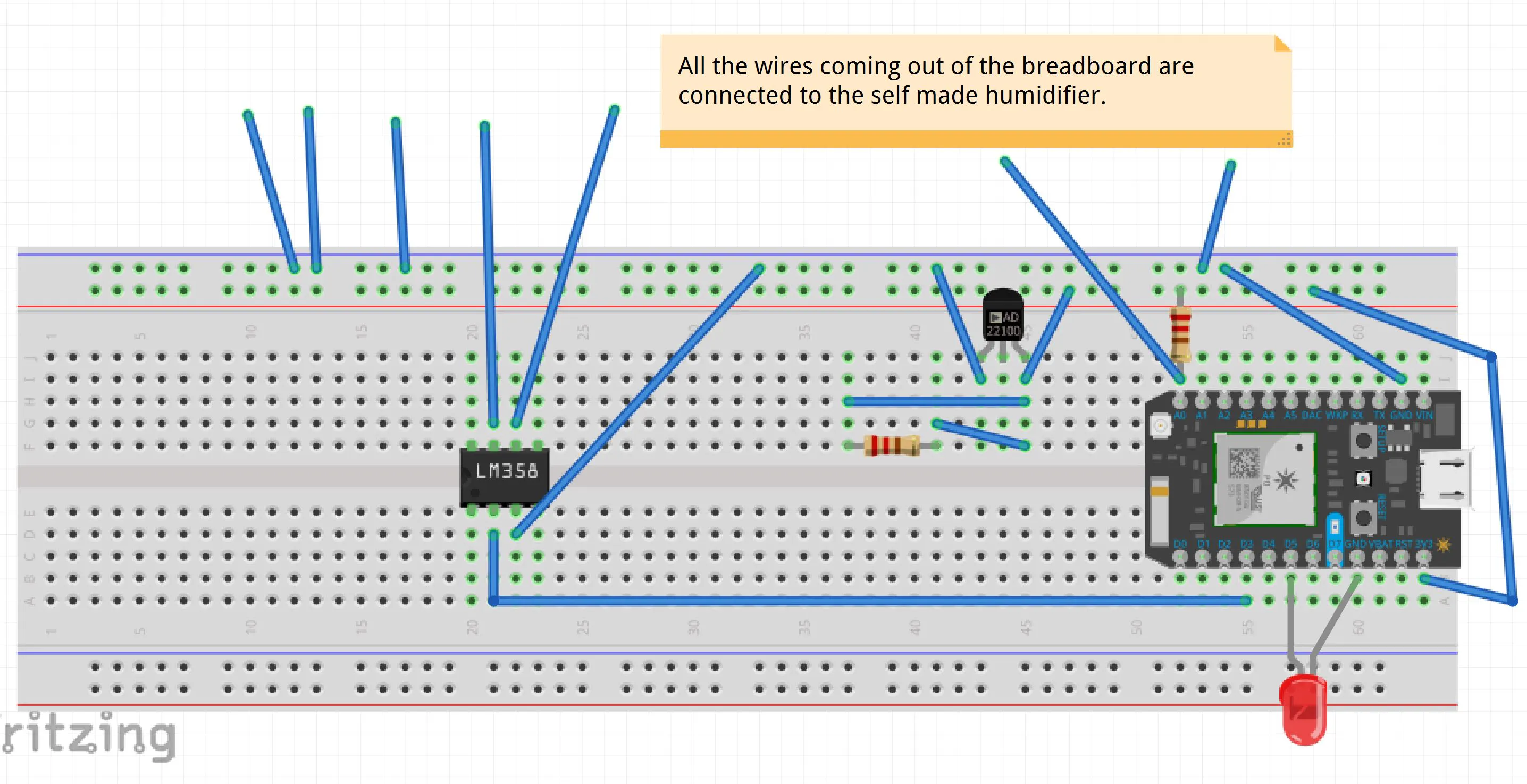### Humidifier Sensor Circuit

This diagram shows the setup for the humidifier. To turn on the heater block, a PWM signal is sent to the relay on the breadboard which then switches the heats inked MOSFET.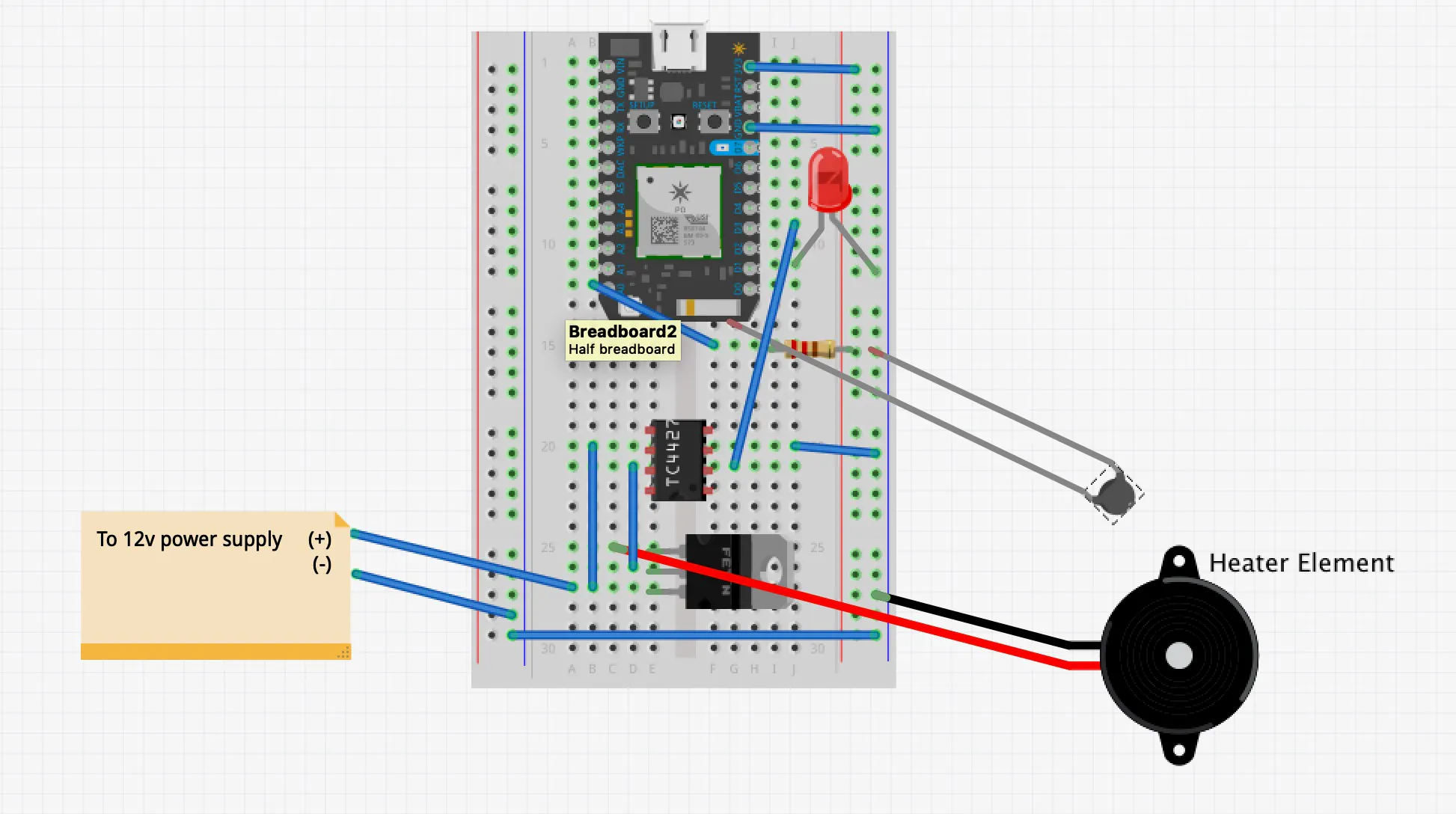## Code

### Working the DHT11 Sensor

C/C++
This code is used to gather the data of the DHT11 sensor and graph it. While sending to the other photon for use.
```// This #include statement was automatically added by the Particle IDE.
#include <PietteTech_DHT.h>

#include "ThingSpeak.h"

#define DHTTYPE  DHT11      // Sensor type DHT11/21/22/AM2301/AM2302
#define DHTPIN   3          // Digital pin for communications

PietteTech_DHT DHT(DHTPIN, DHTTYPE);

int n=0;      // counter
int LEDpin=D6;
float h_set=90;

bool humid = false;

TCPClient client;

unsigned long myChannelNumber = 758578;
const char * myWriteAPIKey = "J9R2AAQEJK14M5W9";

void setup() {
ThingSpeak.begin(client);

Serial.begin(9600);
pinMode (D3, INPUT);
pinMode (LEDpin,OUTPUT);

bool humid = false;

Particle.publish("humiditysensorthreshold", "off");
Particle.subscribe("heaterblockon", myHandler);

}

void loop() {
int result = DHT.acquireAndWait(1000); // wait up to 1 sec (default indefinitely)

switch (result) {
case DHTLIB_OK:
Serial.println("OK");
break;
case DHTLIB_ERROR_CHECKSUM:
Serial.println("Error\n\r\tChecksum error");
break;
case DHTLIB_ERROR_ISR_TIMEOUT:
Serial.println("Error\n\r\tISR time out error");
break;
case DHTLIB_ERROR_RESPONSE_TIMEOUT:
Serial.println("Error\n\r\tResponse time out error");
break;
case DHTLIB_ERROR_DATA_TIMEOUT:
Serial.println("Error\n\r\tData time out error");
break;
case DHTLIB_ERROR_ACQUIRING:
Serial.println("Error\n\r\tAcquiring");
break;
case DHTLIB_ERROR_DELTA:
Serial.println("Error\n\r\tDelta time to small");
break;
case DHTLIB_ERROR_NOTSTARTED:
Serial.println("Error\n\r\tNot started");
break;
default:
Serial.println("Unknown error");
break;
}

n++;

int humidity = DHT.getHumidity();
int temperature = DHT.getFahrenheit();

if(DHT.getHumidity()<h_set){
Particle.publish("humiditysensorthreshold", "on");
}
else {
Particle.publish("humiditysensorthreshold", "off");
}

ThingSpeak.setField(1,humidity);
ThingSpeak.setField(2,temperature);

Serial.print(DHT.getHumidity());
Serial.println("h");
Serial.print(DHT.getFahrenheit());
Serial.println("t");

ThingSpeak.writeFields(myChannelNumber, myWriteAPIKey);

delay(15000); // ThingSpeak will only accept updates every 15 seconds.
}

void myHandler(String event, String data)
{
if(data == "on"){
digitalWrite(LEDpin,HIGH);}
if(data == "off"){
digitalWrite(LEDpin,LOW);}
}
```

### Working the Humidifer

C/C++
This code is used to start and stop the humidifier based on the data received from the other photon.
```#include <math.h>
int ThermistorPin = A0;
int PWM_Pin = D3;
int LEDpin= D5;

int EState;
int ELastState;
int counter=0;

int Resistor1 = 10000;
int B=3950;// thermistor beta coefficient
int samples;
int i;
float Therm_resistance;
double T;
float Vout;

float kp = 6;
float ki = 0.5;
float PID_p=0;    float PID_i=0;
float PID_error=0;
float PID_value=0;

int set_temperature = 220;

float previous_error;
float elapsedTime, timePrev;

void setup() {
Particle.publish("heaterblockon", "off");
Particle.subscribe("humiditysensorthreshold", myHandler);
Serial.begin(9600);

pinMode (PWM_Pin, OUTPUT);
pinMode (ThermistorPin,INPUT);
pinMode (LEDpin, OUTPUT);
digitalWrite(LEDpin,LOW);

}

void loop() {

for (i=0; i< 8; i++) {
delay(10);
}

// average all the samples out
Vout = 0;
for (i=0; i< 8; i++) {
Vout += samples[i];
}
Vout /= 8;

//To find thermistor resistance
Therm_resistance = Resistor1 / (4095/Vout-1);
//Steinhart_Hart equation, ouputs in kelvin
T = (1/(((log(Therm_resistance / 10000))/B)+(1.0 / (25 + 273.15))));
//convert to celcius
T = T - 273.15;
//convert to farenheit
T = (T * 9.0)/ 5.0 + 32.0;

//calculate the error
PID_error = set_temperature - T;

//calculations needed for PID_p
PID_p=PID_error;

//Calculate the I value
PID_i += (PID_error);
if (PID_i>50){
PID_i=50;
}
if (PID_i<-50){
PID_i=-50;
}
else{
PID_i=PID_i;
}

//Final total PID value
PID_value = (PID_p*kp) + (PID_i*ki);

//PWM range between 0 and 255 (0-100 duty cycle)
if(PID_value < 0)
{    PID_value = 0;    }
if(PID_value > 255)
{    PID_value = 255;  }
else PID_value=PID_value;

{analogWrite(PWM_Pin,PID_value);
//digitalWrite(LEDpin, HIGH);}
}
previous_error = PID_error;

Serial.print(PID_value);
Serial.println("pid");
Serial.print(set_temperature);
Serial.println("tset");
Serial.print(PID_i);
Serial.println("i");
Serial.print(set_temperature);
Serial.println("t");

delay(1000);
}

void myHandler(String event, String data)
{
if(data == "on"){
Particle.publish("heaterblockon", "on");
set_temperature=220;
digitalWrite(LEDpin,HIGH);}
if(data == "off"){
Particle.publish("heaterblockon", "off");
set_temperature=0;
digitalWrite(LEDpin,LOW);}
}
```

## Credits

### John Ranshaw

1 project • 0 followers

### Christopher Ames

1 project • 0 followers